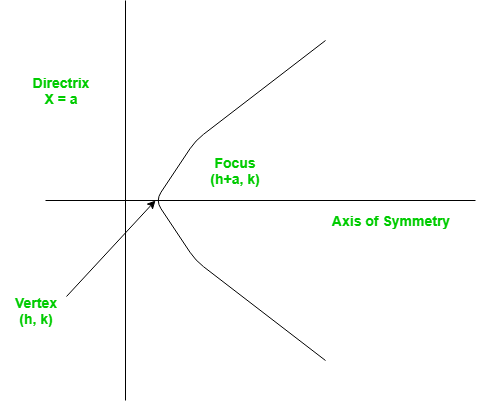# Finding the vertex, focus and directrix of a parabola

• Difficulty Level : Basic
• Last Updated : 22 Jun, 2022

Problem – Find the vertex, focus and directrix of a parabola when the coefficients of its equation are given.
A set of points on a plain surface that forms a curve such that any point on that curve is equidistant from the focus is a parabola.
Vertex of a parabola is the coordinate from which it takes the sharpest turn whereas a is the straight line used to generate the curve.The standard form of a parabola equation is. Given the values of a, b and c; our task is to find the coordinates of vertex, focus and the equation of the directrix.
Example –

```Input : 5 3 2
Output : Vertex:(-0.3, 1.55)
Focus: (-0.3, 1.6)
Directrix: y=-198
Consult the formula below for explanation.```

This problem is a simple example of implementations of formulae. Given below are the required set of formulae which will help us tackle the problem.

`For a parabola in the formVertex:Focus:Directrix:`

## C++

 `#include ``using` `namespace` `std;` `// Function to calculate Vertex, Focus and Directrix``void` `parabola(``float` `a, ``float` `b, ``float` `c)``{``    ``cout << ``"Vertex: ("` `<< (-b / (2 * a)) << ``", "``         ``<< (((4 * a * c) - (b * b)) / (4 * a))``         ``<< ``")"` `<< endl;``    ``cout << ``"Focus: ("` `<< (-b / (2 * a)) << ``", "``         ``<< (((4 * a * c) - (b * b) + 1) / (4 * a))``         ``<< ``")"` `<< endl;``    ``cout << ``"Directrix: y="``         ``<< c - ((b * b) + 1) * 4 * a << endl;``}` `// Driver Function``int` `main()``{``    ``float` `a = 5, b = 3, c = 2;``    ``parabola(a, b, c);``    ``return` `0;``}`

## Java

 `// Java program to find the vertex,``// focus and directrix of a parabola` `class` `GFG {``    ` `    ``// Function to calculate Vertex,``    ``// Focus and Directrix``    ``static` `void` `parabola(``float` `a,``                         ``float` `b, ``float` `c)``    ``{``        ` `        ``System.out.println(``"Vertex: ("` `+``                          ``(-b / (``2` `* a)) + ``", "` `+``                          ``(((``4` `* a * c) - (b * b)) /``                          ``(``4` `* a)) + ``")"``);``                    ` `        ``System.out.println(``"Focus: ("` `+``                          ``(-b / (``2` `* a)) + ``", "`    `+``                          ``(((``4` `* a * c) - (b * b) + ``1``) /``                          ``(``4` `* a)) + ``")"``);``            ` `        ``System.out.println(``"Directrix:"` `+ ``" y="` `+``                          ``(``int``)(c - ((b * b) + ``1``) *``                          ``4` `* a));``    ``}` `    ``// Driver Function``    ``public` `static` `void` `main(String[] args)``    ``{``        ``float` `a = ``5``, b = ``3``, c = ``2``;``        ` `        ``// Function calling``        ``parabola(a, b, c);``    ``}``}` `// This code is contributed by``// Smitha Dinesh Semwal`

## Python 3

 `# Function to calculate Vertex,``# Focus and Directrix``def` `parabola(a, b, c):` `    ``print``(``"Vertex: ("` `, (``-``b ``/` `(``2` `*` `a)),``        ``", "``, (((``4` `*` `a ``*` `c) ``-` `(b ``*` `b))``            ``/` `(``4` `*` `a)), ``")"``, sep ``=` `"")``              ` `    ``print``(``"Focus: ("` `, (``-``b ``/` `(``2` `*` `a)),``    ``", "``, (((``4` `*` `a ``*` `c) ``-` `(b ``*` `b) ``+` `1``)``            ``/` `(``4` `*` `a)), ``")"``, sep ``=` `"")``               ` `    ``print``(``"Directrix: y="``, c ``-` `((b ``*` `b)``                ``+` `1``) ``*` `4` `*` `a, sep ``=` `"")` `# Driver Function``a ``=` `5``b ``=` `3``c ``=` `2``parabola(a, b, c)` `# This code is contributed by Smitha.`

## C#

 `// C# program to find the vertex,``// focus and directrix of a parabola``using` `System;` `class` `GFG {``    ` `    ``// Function to calculate Vertex,``    ``// Focus and Directrix``    ``static` `void` `parabola(``float` `a,``                         ``float` `b, ``float` `c)``    ``{``        ``Console.WriteLine(``"Vertex: ("` `+``                         ``(-b / (2 * a)) + ``", "` `+``                         ``(((4 * a * c) - (b * b)) /``                         ``(4 * a)) + ``")"``);``                    ` `        ``Console.WriteLine(``"Focus: ("` `+``                         ``(-b / (2 * a)) + ``", "` `+``                         ``(((4 * a * c) - (b * b) + 1) /``                         ``(4 * a)) + ``")"``);``                ` `        ``Console.Write(``"Directrix:"` `+ ``" y="` `+``                     ``(``int``)(c - ((b * b) + 1) * 4 * a));``    ``}` `    ``// Driver Function``    ``public` `static` `void` `Main()``    ``{``        ``float` `a = 5, b = 3, c = 2;``        ` `        ``// Function calling``        ``parabola(a, b, c);``    ``}``}` `// This code is contributed by nitin mittal`

## PHP

 ``

## Javascript

 ``

Output –

```Vertex:(-0.3, 1.55)
Focus: (-0.3, 1.6)
Directrix: y=-198```

Time Complexity: O(1)

Auxiliary Space: O(1)

My Personal Notes arrow_drop_up